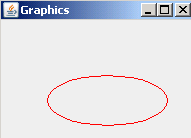Ads

Tutorials

# Java get Graphics

In the given example, we have used the AWT and Swing package to used the method getGraphics(). For this, we have used the class BufferedImage. The method image.getGraphics() returns the Graphics object.

In the given example, we have used the AWT and Swing package to used the method getGraphics(). For this, we have used the class BufferedImage. The method image.getGraphics() returns the Graphics object.

# Java get Graphics

In this section you will learn how to use the method getGraphics().

In the given example, we have used the AWT and Swing package to used the method getGraphics(). For this, we have used the class BufferedImage. The method image.getGraphics() returns the Graphics object. The method graphics.drawOval(150,50,120,50) draws an oval on the frame and the method graphics.setColor(Color.red) sets the color of the oval.

Here is the code of GetGraphics.java

 import java.awt.*; import javax.swing.*; import java.awt.image.BufferedImage; class GetGraphics {   public static void main(String[] args)   {   JFrame frame=new JFrame();   frame.setLayout(new FlowLayout());   BufferedImage image=new BufferedImage(400,400,BufferedImage.TYPE_INT_ARGB);   Graphics graphics=image.getGraphics();      graphics.setColor(Color.red);   graphics.drawOval(150,50,120,50);   graphics.dispose();   JLabel label=new JLabel(new ImageIcon(image));   frame.add(label);   frame.setTitle("Graphics");   frame.setSize(200,150);   frame.setVisible(true);   } }

Output will be displayed as:Download Source Code

Ads

Ads